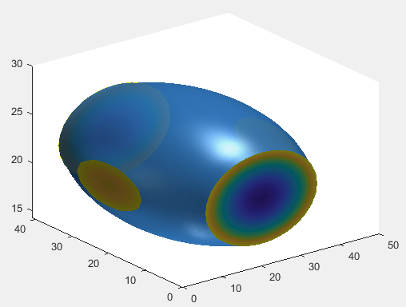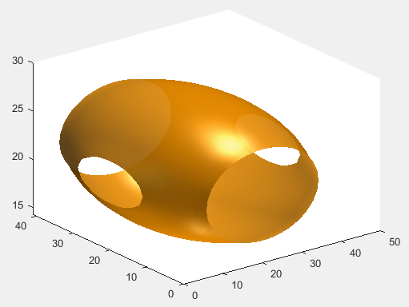Managing Properties of Chart Classes

When you develop a custom chart as a subclass of the ChartContainer base class, you can use certain techniques to make your code more robust, efficient, and tailored to the needs of your users. These techniques focus on how you define and manage the properties of your class. Use any that are helpful for the type of visualization you want to create and the user experience you want to provide.

• Initialize property values — Set the default state of the chart in case your users call the implicit constructor without any input arguments.

• Validate property values — Ensure that the values are valid before using them to perform a calculation or configure one of the underlying graphics objects in your chart.

• Customize the property display — Provide a customized list of properties when a user references the chart object without semicolon.

• Optimize the update method — Improve the performance of the update method when only a subset of your properties are used in a time-consuming calculation.

Initialize Property Values

Assign default values for all of the public properties of your class. Doing so configures a valid chart if the user omits some of the name-value pair arguments when they call the constructor method.

For properties that store coordinate data, set the initial values to NaN values or empty arrays so that the default chart is empty when the user does not specify the coordinates. Choose the default coordinates according to the requirements of the plotting functions you plan to call in your class methods. To learn about the requirements, see the documentation for the plotting functions you plan to use.

Validate Property Values

A good practice is to verify the values of your class properties before your code uses those values. A convenient way to do this is to validate the size and class of the properties as you define them. For example, this property block validates the size and class of four properties.

properties
IsoValue (1,1) double = 0.5
Enclose {mustBeMember(Enclose,{'above','below'})} = 'below'
CapVisible (1,1) matlab.lang.OnOffSwitchState = 'on'
Color (1,3) double {mustBeGreaterThanOrEqual(Color,0),...
mustBeLessThanOrEqual(Color,1)} = [.2 .5 .8]
end

• IsoValue must be a 1-by-1 array of class double.

• Enclose must have a value of either 'above' or 'below'.

• CapVisible must be a 1-by-1 array of class matlab.lang.OnOffSwitchState.

• Color must be a 1-by-3 array of class double, where each value is in the range [0,1].

You can also validate properties that store the underlying graphics objects in your chart. To determine the class name of an object, call the corresponding plotting function at the command line, and then call the class function to get the class name. For example, if you plan to call the patch function in your setup method, call the patch function at the command line with an output argument (the input arguments do not matter). Then pass the output to the class function to get its class name.

x = patch(NaN,NaN,NaN);
class(x)
ans =

'matlab.graphics.primitive.Patch'

Use the output of the class function to validate the class for the corresponding property in your class. For example, each of the following properties stores a Patch object.

properties (Access = private,Transient,NonCopyable)
IsoPatch (1,1) matlab.graphics.primitive.Patch
CapPatch (1,1) matlab.graphics.primitive.Patch
end

Occasionally, you might want to define a property that can store different shapes and classes of values. For example, if you define a property that can store a character vector, cell array of character vectors, or string array, omit the size and class validation or use a custom property validation method.

Customize the Property Display

One of the benefits of defining your chart as a subclass of the ChartContainer base class is that it also inherits from the matlab.mixin.CustomDisplay class. Thus, you can customize the list of properties MATLAB® displays in the Command Window when you reference the chart without a semicolon. To customize the property display, overload the getPropertyGroups method. Within that method, you can customize which properties are listed and the order of the list. For example, consider an IsoSurfCapChart class that has the following public properties.

properties
IsoValue (1,1) double = 0.5
Enclose {mustBeMember(Enclose,{'above','below'})} = 'below'
CapVisible (1,1) matlab.lang.OnOffSwitchState = 'on'
Color (1,3) double {mustBeGreaterThanOrEqual(Color,0),...
mustBeLessThanOrEqual(Color,1)} = [.2 .5 .8]
end

The following getPropertyGroups method specifies the scalar object property list as Color, IsoValue, Enclose, and CapVisible.

function propgrp = getPropertyGroups(obj)
if ~isscalar(obj)
% List for array of objects
propgrp = getPropertyGroups@matlab.mixin.CustomDisplay(obj);
else
% List for scalar object
propList = {'Color','IsoValue','Enclose','CapVisible'};
propgrp = matlab.mixin.util.PropertyGroup(propList);
end
end

When the user references an instance of this chart without a semicolon, MATLAB displays the customized list.

c = IsoSurfCapChart
c =

IsoSurfCapChart with properties:

Color: [0.2000 0.5000 0.8000]
IsoValue: 0.5000
Enclose: 'below'
CapVisible: on

Optimize the update Method

In most cases, the update method of your class reconfigures all the relevant aspects of your chart that depend on the public properties. Sometimes, the reconfiguration involves an expensive calculation that is time consuming. If the calculation involves only a subset of the properties, you can design your class to execute that code only when it is necessary.

One way to optimize the update method is to add these components to your class:

• Define a private property called ExpensivePropChanged that accepts a logical value. This property indicates whether any of the properties used in the expensive calculation have changed.

• Write a set method for each property involved in the expensive calculation. Within each set method, set the ExpensivePropChanged property to true.

• Write a protected method that performs the expensive calculation.

• Write a conditional statement in the update method that checks the value of ExpensivePropChanged. If the value is true, execute the method that performs the expensive calculation.

The following code provides a simplified implementation of this design.

classdef OptimizedChart < matlab.graphics.chartcontainer.ChartContainer

properties
Prop1
Prop2
end
properties(Access=private,Transient,NonCopyable)
ExpensivePropChanged (1,1) logical = true
end

methods(Access = protected)
function setup(obj)
% Configure chart
% ...
end
function update( obj )
% Perform expensive computation if needed
if obj.ExpensivePropChanged
doExpensiveCalculation(obj);
obj.ExpensivePropChanged = false;
end

% Update other aspects of chart
% ...
end
function doExpensiveCalculation(obj)
% Expensive code
% ...
end
end

methods
function set.Prop2(obj,val)
obj.Prop2 = val;
obj.ExpensivePropChanged = true;
end
end
end
In this case, Prop2 is involved in the expensive calculation. The set.Prop2 method sets the value of Prop2, and then it sets ExpensivePropChanged to true. Thus, the next time the update method runs, it calls doExpensiveCalculation only if ExpensivePropChanged is true. Then the update method continues to update other aspects of the chart.

Example: Optimized Isosurface Chart with Customized Property Display

Define an IsoSurfCapChart class for displaying an isosurface with the associated isocaps. Include the following features:

• Properties that use size and class validation

• A customized property display

• An optimized update method that recalculates the isosurface and isocaps only if one or more of the relevant properties changed

To define this class, create a program file named IsoSurfCapChart.m in a folder that is on the MATLAB path. Then implement the class by following the steps in the table.

StepImplementation

Derive from the ChartContainer base class.

classdef IsoSurfCapChart < matlab.graphics.chartcontainer.ChartContainer

Define the public properties using class and size validation.

• VolumeData, IsoValue, and Color are parameters for the isosurface.

• Enclose, WhichCapPlane, and CapVisible are parameters for the isocaps.

properties
VolumeData double = rand(25,25,25)
IsoValue (1,1) double = 0.5
Enclose {mustBeMember(Enclose,{'above','below'})} = 'below'
WhichCapPlane {mustBeMember(WhichCapPlane,{'all','xmin',...
'xmax','ymin','ymax','zmin','zmax'})} = 'all'
CapVisible (1,1) matlab.lang.OnOffSwitchState = 'on'
Color (1,3) double {mustBeGreaterThanOrEqual(Color,0),...
mustBeLessThanOrEqual(Color,1)} = [.2 .5 .8]
end

Define the private properties.

• IsoPatch and CapPatch store the Patch objects for the isosurface and isocaps.

• SmoothData stores a smoothed version of the volume data.

• ExpensivePropChanged indicates whether the update method needs to recalculate the isosurface and isocaps.

properties(Access = private,Transient,NonCopyable)
IsoPatch (1,1) matlab.graphics.primitive.Patch
CapPatch (1,1) matlab.graphics.primitive.Patch
SmoothData double = [];
ExpensivePropChanged (1,1) logical = true
end

Implement the setup method. In this case, call the patch function twice to create the Patch objects for the isosurface and isocaps. Store the objects in the corresponding properties, and configure the axes.

methods(Access = protected)
function setup(obj)
ax = getAxes(obj);

% Create two Patch objects
obj.IsoPatch = patch(ax,NaN,NaN,NaN, 'EdgeColor', 'none', ...
'FaceColor',[.2 .5 .8],'FaceAlpha',0.9);
hold(ax,'on');
obj.CapPatch = patch(ax,NaN,NaN,NaN,'EdgeColor', 'none', ...
'FaceColor','interp');

% Configure the axes
view(ax,3)
camlight(ax, 'infinite');
camlight(ax,'left');
lighting(ax, 'gouraud');
hold(ax,'off');
end

Implement the update method. Decide whether to call the doExpensiveCalculation method by testing the value of ExpensivePropChanged. Then continue updating the other (less expensive) aspects of the chart.

function update(obj)
% Perform expensive computation if needed
if obj.ExpensivePropChanged
doExpensiveCalculation(obj);
obj.ExpensivePropChanged = false;
end

% Update visibility of CapPatch and update color
obj.CapPatch.Visible = obj.CapVisible;
obj.IsoPatch.FaceColor = obj.Color;
end

Implement the doExpensiveCalculation method, which smooths the volume data and recalculates the faces and vertices of the isosurface and isocaps.

function doExpensiveCalculation(obj)
% Update isosurface
obj.SmoothData = smooth3(obj.VolumeData,'box',7);
[F,V] = isosurface(obj.SmoothData, obj.IsoValue);
set(obj.IsoPatch,'Faces',F,'Vertices',V);
isonormals(obj.SmoothData,obj.IsoPatch);

% Update isocaps
[m,n,p] = size(obj.SmoothData);
[Xc,Yc,Zc] = meshgrid(1:n,1:m,1:p);
[Fc,Vc,Cc] = isocaps(Xc,Yc,Zc,obj.SmoothData,obj.IsoValue,...
obj.Enclose,obj.WhichCapPlane);
set(obj.CapPatch,'Faces',Fc,'Vertices',Vc,'CData',Cc);
end

Implement the getPropertyGroups method to customize the property display.

function propgrp = getPropertyGroups(obj)
if ~isscalar(obj)
% List for array of objects
propgrp = getPropertyGroups@matlab.mixin.CustomDisplay(obj);

else
% List for scalar object
propList = {'Color','IsoValue','Enclose','CapVisible',...
'WhichCapPlane','VolumeData'};
propgrp = matlab.mixin.util.PropertyGroup(propList);
end
end
end

Implement the set methods for each expensive property (VolumeData, IsoValue, and Enclose). Within each method, set the corresponding property value, and then set ExpensivePropChanged to true.

methods
function set.VolumeData(obj,val)
obj.VolumeData = val;
obj.ExpensivePropChanged = true;
end
function set.IsoValue(obj, val)
obj.IsoValue = val;
obj.ExpensivePropChanged = true;
end
function set.Enclose(obj, val)
obj.Enclose = val;
obj.ExpensivePropChanged = true;
end
end
end

Next, create an array of volume data, and then create an instance of IsoSurfCapChart.

[X,Y,Z] = meshgrid(-2:0.1:2);
v = (1/9)*X.^2 + (1/16)*Y.^2 + Z.^2;
c = IsoSurfCapChart('VolumeData',v,'IsoValue',0.5)
c =

IsoSurfCapChart with properties:

Color: [0.2000 0.5000 0.8000]
IsoValue: 0.5000
Enclose: 'below'
CapVisible: on
WhichCapPlane: 'all'
VolumeData: [41×41×41 double]Change the color of c and hide the isocaps.

c.Color = [1 0.60 0];
c.CapVisible = false;